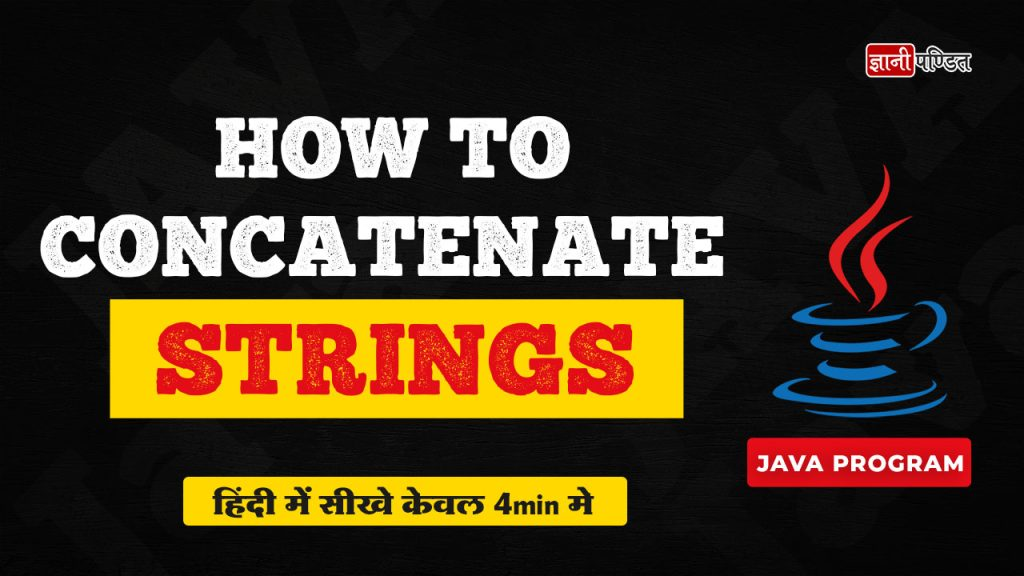# Java Tutorial## Java Program to Find Smallest Number in an Array

In this article, we are going to understand and implement a simple Java program, in which we are given an array, and we need to find the smallest number in the array. The Java program is very simple to do, provided that you are familiar with how to deal with arrays, and you know a …## Factorial of a Number Using Recursion in Java

At times, you might be required to calculate the factorial of a given number, so, in this article, we are going to learn about the simple program in Java, through which we can easily calculate the factorial of the given number. We will not only see the program but also the logic behind calculating the …

## What is Java?

When it comes to programming, there are many different programming languages that are used for different purposes. Many people in this world, always have their eyes on the trend, and on the most popular and most widely used programming languages in this world. One such very widely used, and very popular programming language is Java. …## How to Concatenate Strings in Java

In this video, we are going to learn about how can we concatenate strings in Java. At times, In our Java programs, we might require to concatenate strings, and in such situations, we can concatenate the strings by doing some simple stuff. We can make use of the + operator with the strings, to concatenate …## Convert Celsius to Fahrenheit Program in Java

Here, we are performing a simple Java program, to convert the temperature from degree Celsius to degree Fahrenheit. To convert the temperature from degree Celsius to degree Fahrenheit, we just need to make use of a simple conversion formula. You can also try to perform this simple program, and many such others simple conversion programs, …

## Java Program to Find Largest Number in an Array

In this article, we are going to understand and perform a simple task in Java, in which, we are given an array, and we need to find the largest number in the array. We are required to write a Java program for the same. So, we will first understand the logic behind the program, and …

## How to find the first and last digit of a number in Java

In this article, we are going to understand and perform a simple Java program, in which, we are going to find the first and last digits of a given number. For example, the number we have is 1234, so the first and last digits that we need to have here are 1 and 4 respectively. …